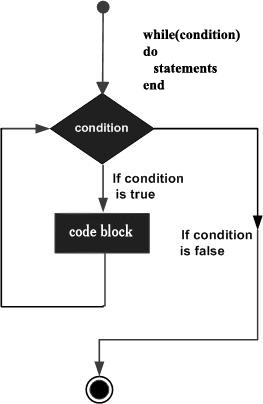# Lua - while Loop

A while loop statement in Lua programming language repeatedly executes a target statement as long as a given condition is true.

## Syntax

The syntax of a while loop in Lua programming language is as follows −

```while(condition)
do
statement(s)
end
```

Here, statement(s) may be a single statement or a block of statements. The condition may be any expression, and true is any non-zero value. The loop iterates while the condition is true.

When the condition becomes false, the program control passes to the line immediately following the loop.

## Flow DiagramHere, the key point to note is that the while loop might not be executed at all. When the condition is tested and the result is false, the loop body will be skipped and the first statement after the while loop will be executed.

## Example

```a = 10

while( a < 20 )
do
print("value of a:", a)
a = a+1
end
```

When the above code is built and executed, it produces the following result −

```value of a:	10
value of a:	11
value of a:	12
value of a:	13
value of a:	14
value of a:	15
value of a:	16
value of a:	17
value of a:	18
value of a:	19
```
lua_loops.htm